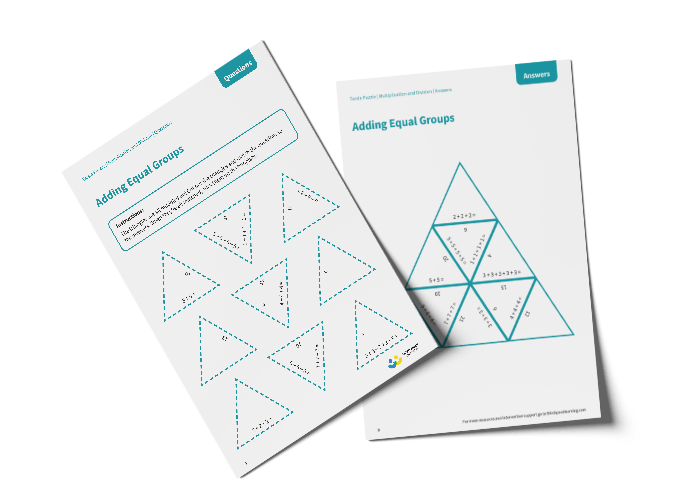# Tarsia Puzzle Adding Equal Groups (Year 1)

The triangles in the tarsia puzzle have all been mixed up. In this adding equal groups tarsia puzzle, pupils will need to match the repeated addition question to the correct answer to create one large triangle.

This Year 1 adding equal groups tarsia puzzle worksheet contains:

• One question sheet
• One template sheet

Year 1

Multiplication